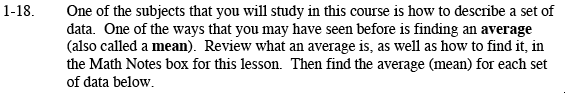### Home > MC2 > Chapter 1 > Lesson 1.1.3 > Problem1-18

1-18.$82,64,73,91$

To find the average (mean), add all the values together and divide by the total number of values.

Find 82 + 64 + 73 + 91 + 85

Divide this total by the number of data values, in this case 5.

79

$0,1,3,2$

To find the average, calculate:

$: 35,40,12,16,25,10$

Look at the strategies in parts (a) and (b).

Explore using the etool below.
Click the link at right for the full version of the eTool: MC2 1-18HW eTool